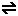# Kpc

Different solutes will have different solubilities in different solvents. If a solute is dissolved in a mixture of two immiscible liquid phases, the partition will involve the distribution of a solute between two immiscible liquid phases. As with our basics learnt so far from Kc as a basis, we will simply use Kpc to represent the Partition Coefficient for a given solute dissolved in a mixture of two immiscible liquid phases.

For a given solute X:

 Kpc = [X(liquid 1)] —————- [X(liquid 2)]

Kpc has no units and is temperature dependent.

If more of the substance X is added to the system, the solute will distribute itself between the immiscible liquids so that the ratio of the solute concentrations remains the same at constant temperature independently of the total quantity of X in the same molecular state, and that is essentially the partition equilibrium law.

• Examples of partition systems involving two immiscible liquids

1. Iodine in water/tetrachloromethane: I2(aq)I2(CCl4) : K = [I2(aq)] / [I2(CCl4)] = 0.0116

• The non-polar iodine is much more soluble in the non-polar organic solvent than in the highly polar water solvent. The iodine cannot disrupt few of the strong intermolecular forces of hydrogen bonding between water molecules.

• This system can be analysed by titrating extracted aliquots with standardised sodium thiosulphate and starch indicator.

• If the aqueous solution is replaced by aqueous potassium iodide, the simple Kpartition expression does not hold because of a 2nd homogeneous equilibrium and both equilibria expressions must be satisfied:

• I(aq) + I2(aq)I3(aq) as well as I2(aq)I2(CCl4)

2. Ammonia in water/trichloromethane: NH3(aq)NH3(CHCl3) : K = [NH3(aq)] / [NH3(CHCl3)] = 26

• The highly polar ammonia can hydrogen bond with the even more polar water molecules, hence ammonia’s much greater solubility in water than the less polar trichloromethane.

• This system can be analysed by titrating extracted aliquots with standardised hydrochloric acid and methyl orange indicator.

• The small % of the highly soluble ammonia that ionises in water can be reasonably ignored in this case.

• Example calculations:

• Ex. Q4.1.1 20g of butanedioic acid (BDA) was shaken with a mixture of 100 cm3 ether and 100 cm3 water at 25oC. After titration with standard sodium hydroxide the concentration of the acid was found to be 0.024 mol dm-3 in ether and 0.16 mol dm-3 in water.

• (a) Calculate the distribution coefficient KD for butanedioic acid between ether/water.

• KD = [BDA(ether)] / [BDA(water)] = 0.024 / 0.16 = 0.15

• (b) If 10g of BDA had been shaken with 50 cm3 of each solvent at 25oC, what value would expect for KD if the layers were again analysed?

• 0.15, since the partition law states the ratio of concentrations remains constant at constant temperature.

• (c) If 10g of butanedioic acid was dissolved in 50 cm3 of ether at 25oC, calculate how much of the acid can be extracted with 50 cm3 of water.

• If we call x the mass in g extracted into water, V the liquid volumes, and expressing the concentrations in gcm-3, substitution into the partition expression gives …

•  KD = [(10-x)/Vether]       (10-x) ———————- = ——- (since V’s cancel out) [x/Vwater]            x
• Rearranging: 0.15 x = 10 – x, so 1.15x = 10
• therefore x = 10/1.15 = 8.7g of BDA extracted.

Not it is up to us to use the knowledge given to us. Partition coefficients have many extremely critical uses, and I would now invite you students to comment and post some Case Studies that you find useful in our lives.

Maxim Yap
HoD Chemistry 2012